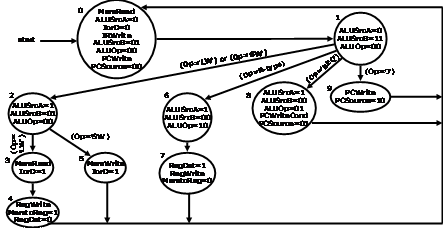Computer Organization and Structure

Homework #4

Due: 2003/12/16

1.      We wish to add the instruction addi (add immediate) to the single-cycle datapath. Add any necessary datapaths and control signals to the following single-cycle datapath:and show the necessary additions to the following table:

 Instruction RegDst ALUSrc Memto Reg Reg Write Mem Read Mem Write Branch ALUOp1 ALUOp2 R-format 1 0 0 1 0 0 0 1 0 lw 0 1 1 1 1 0 0 0 0 sw X 1 X 0 0 1 0 0 0 beq X 0 X 0 0 0 1 0 1

Now, we wish to add the instruction bne (branch if not equal). Add any necessary datapaths and control signals to the above single-cycle datapath and show the necessary additions to the above table.

2.      A friend is proposing that the control signal MemtoReg be eliminated. The multiplexor that has MemtoReg as an input will instead use the control signal MemRead. Will your friend’s modification work? Consider both datapaths. Moreover, determine whether any of the control signals (other than MemtoReg) in the single-cycle implementation can be eliminated and replaced by another existing control signal. Why or why not?

3.      In estimating the performance of the single-cycle implementation, we assumed that only the major functional units had any delay (i.e., the delay of the multiplexors, control unit, PC access, sign extension unit, and wires was considered to be negligible). Assume that we use a different type of adder for simple addition:

Memory units: 2ns

ALU: 2ns

adder for PC + 4: Xns

Register file (read or write): 1ns

1. What would the cycle time be if X=3 and Y=3?
2. What would the cycle time be if X=5 and Y=5?
3. What would the cycle time be if X=1 and Y=8?

4.      Show how the jump register (jr) instruction can be implemented simply by making changes to the following finite state machine. (It may help you to remember that \$0=\$zero=0.)